Author: Oscar Cronquist Article last updated on February 02, 2023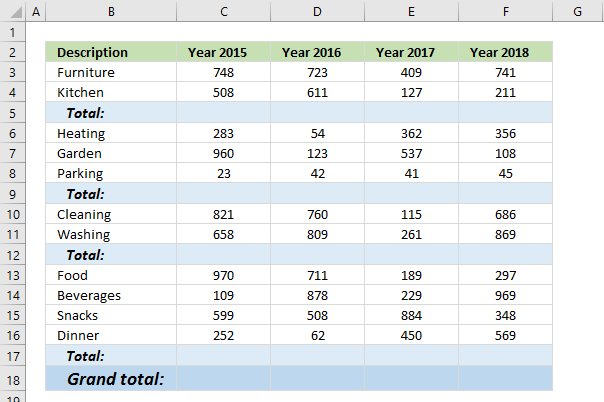This article demonstrates how to find empty cells and populate them automatically with a formula that adds numbers above and returns a total.

Is it possible to quickly select all empty cells and then sum cells above to the next empty cell? Yes, I will show you how.

Can I have a formula in grand total (row 18) that only sums all the totals above? Yes!

## 1. How to select empty cells in a cell range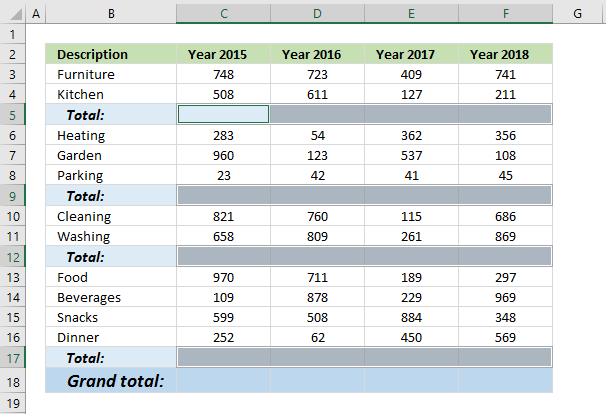The image above demonstrates how to find empty cells in a given cell range.

1. Select all values and the blank total cells.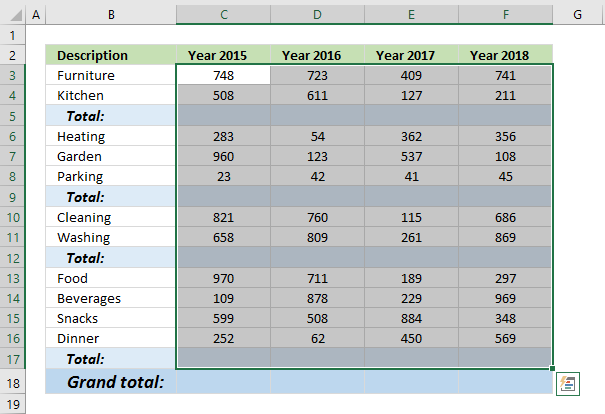2. Press F5, a dialog box appears.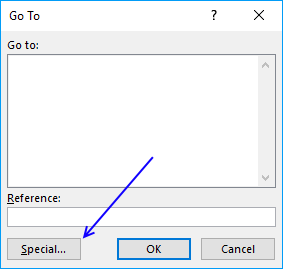3. Press the left mouse on the "Special..." button.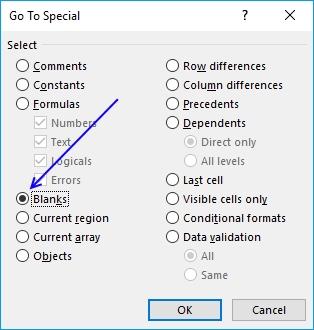4. Press on "Blanks" to select it, then press on the "OK" button.## 2. Populate empty cells with a formula

1. Go to tab "Home" on the ribbon and press with left mouse button on the "AutoSum" button.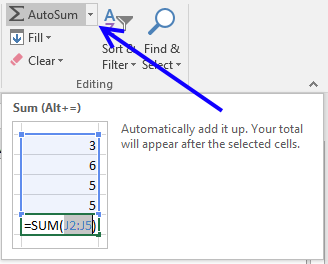2. All empty cells now have a SUM formula that adds all the above values to the next SUM formula.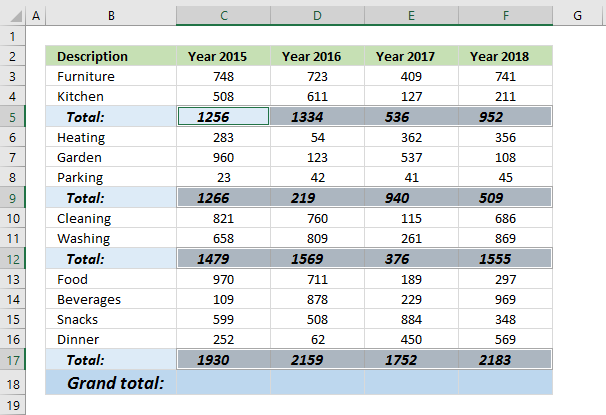## 3. Add grand totals that only sums cells populated with formulas

1. Select cell C18 and type this formula:
=SUMPRODUCT(ISFORMULA(C3:C17)*C3:C17)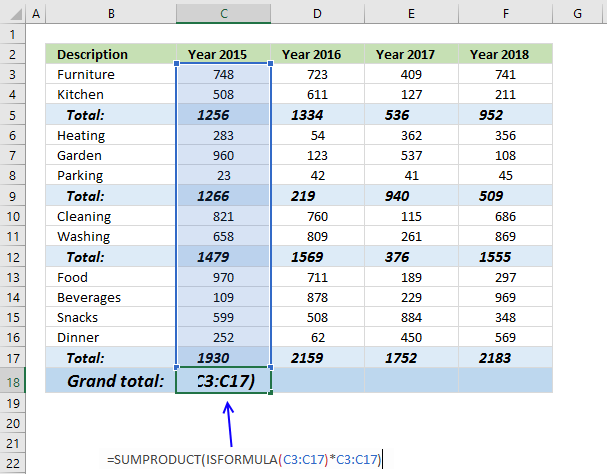2. Press Enter. Copy cell C18 and paste to cell range D18:F18.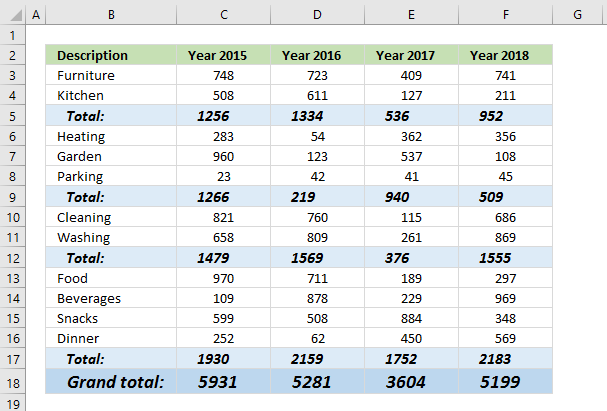### 3.1 Explaining formula in cell C18

#### Step 1 - Check if cell contains a formula

The ISFORMULA function checks if a cell in cell range C3:C17 has a formula. It returns TRUE or FALSE.

ISFORMULA(C3:C17)

returns this array:

{FALSE; FALSE; TRUE; FALSE; FALSE; FALSE; TRUE; FALSE; FALSE; TRUE; FALSE; FALSE; FALSE; FALSE; TRUE}

The picture below shows this array in column D.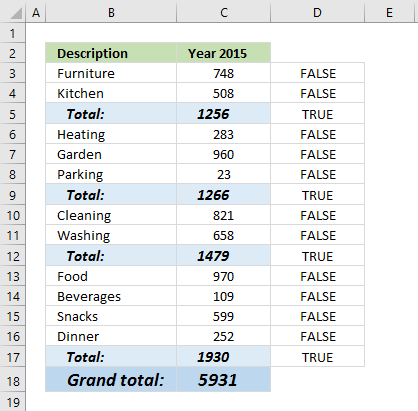The array shows that there is a formula in C5, C9, C12, and C17.

#### Step 2 -  Multiply with value

The asterisk character allows you to multiply numbers and boolean values.

ISFORMULA(C3:C17)*C3:C17 multiplies the boolean values with their corresponding values in column C, shown in column E below.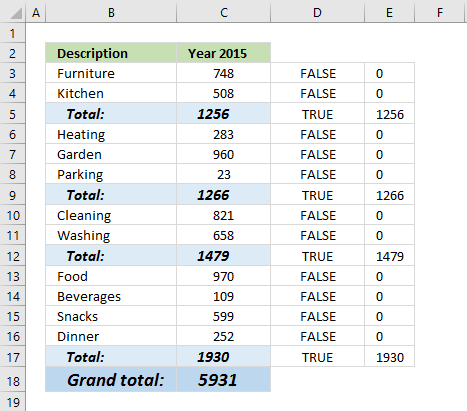ISFORMULA(C3:C17)*C3:C17

becomes

{FALSE; FALSE; TRUE; FALSE; FALSE; FALSE; TRUE; FALSE; FALSE; TRUE; FALSE; FALSE; FALSE; FALSE; TRUE}*C3:C17

becomes

{FALSE; FALSE; TRUE; FALSE; FALSE; FALSE; TRUE; FALSE; FALSE; TRUE; FALSE; FALSE; FALSE; FALSE; TRUE}*{748; 508; 1256; 283; 960; 23; 1266; 821; 658; 1479; 970; 109; 599; 252; 1930}

and returns

{0; 0; 1256; 0; 0; 0; 1266; 0; 0; 1479; 0; 0; 0; 0; 1930}

#### Step 3 - Add values and return the total

The SUMPRODUCT function then sums all values in the array.

SUMPRODUCT(ISFORMULA(C3:C17)*C3:C17)

becomes

SUMPRODUCT({0; 0; 1256; 0; 0; 0; 1266; 0; 0; 1479; 0; 0; 0; 0; 1930})

and returns 5931 in cell C18.

Why not use the SUM function? You need to enter it as an array formula if you use the SUM function. Use the SUM function if you are an Excel 365 user.

## 4. Add grand totals that only sums cells populated with formulas - Excel 2013The following formula won't work, the SUMIF function seems to not be capable of processing the ISFORMULA function. The ISFORMULA function is an Excel 2013 function, they seem incompatible.

=SUMIF(C3:C17, ISFORMULA(C3:C17))

Let me know if you have a solution that allows me to use the SUMIF function.

## 5. Add grand totals that only sums cells populated with formulas - Excel 365=SUM(FILTER(C3:C17, ISFORMULA(C3:C17)))

### 5.1 Explaining formula

#### Step 1 - Check if cell contains formula

The ISFORMULA function returns a boolean value TRUE or FALSE if a cell contains a formula or not.

ISFORMULA(C3:C17)

#### Step 2 - Filter numbers based on boolean values

FILTER(C3:C17,ISFORMULA(C3:C17))

#### Step 3 - Add numbers and return a total

SUM(FILTER(C3:C17,ISFORMULA(C3:C17)))

### Get the Excel fileFind-empty-cells-and-sum-cells-abovev2.xlsx